# Calculus 3 : Normal Vectors

## Example Questions

### Example Question #11 : Normal Vectors

Determine whether the two vectors,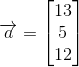and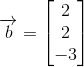, are orthogonal or not.

The two vectors are not orthogonal.

The two vectors are orthogonal.

The two vectors are orthogonal.

Explanation:

Vectors can be said to be orthogonal, that is to say perpendicular or normal, if their dot product amounts to zero:To find the dot product of two vectors given the notation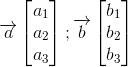Simply multiply terms across rows: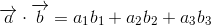For our vectors,and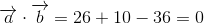The two vectors are orthogonal.

### Example Question #12 : Normal Vectors

Determine whether the two vectors,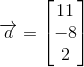and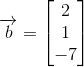, are orthogonal or not.

The two vectors are orthogonal.

The two vectors are not orthogonal.

The two vectors are orthogonal.

Explanation:

Vectors can be said to be orthogonal, that is to say perpendicular or normal, if their dot product amounts to zero: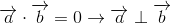To find the dot product of two vectors given the notationSimply multiply terms across rows:For our vectors,and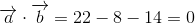The two vectors are orthogonal.

### Example Question #13 : Normal Vectors

Determine whether the two vectors,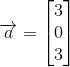and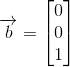, are orthogonal or not.

The two vectors are orthogonal.

The two vectors are not orthogonal.

The two vectors are not orthogonal.

Explanation:

Vectors can be said to be orthogonal, that is to say perpendicular or normal, if their dot product amounts to zero:To find the dot product of two vectors given the notationSimply multiply terms across rows:For our vectors,and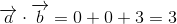The two vectors are not orthogonal.

### Example Question #14 : Normal Vectors

Determine whether the two vectors,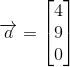and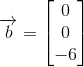, are orthogonal or not.

The two vectors are orthogonal.

The two vectors are not orthogonal.

The two vectors are orthogonal.

Explanation:

Vectors can be said to be orthogonal, that is to say perpendicular or normal, if their dot product amounts to zero:To find the dot product of two vectors given the notationSimply multiply terms across rows:For our vectors,and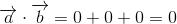The two vectors are orthogonal.

### Example Question #15 : Normal Vectors

Determine whether the two vectors,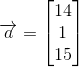and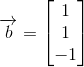, are orthogonal or not.

The two vectors are orthogonal.

The two vectors are not orthogonal.

The two vectors are orthogonal.

Explanation:

Vectors can be said to be orthogonal, that is to say perpendicular or normal, if their dot product amounts to zero:To find the dot product of two vectors given the notationSimply multiply terms across rows:For our vectors,and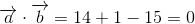The two vectors are orthogonal.

### Example Question #16 : Normal Vectors

Determine whether the two vectors,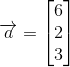and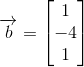, are orthogonal or not.

The two vectors are not orthogonal.

The two vectors are orthogonal.

The two vectors are not orthogonal.

Explanation:

Vectors can be said to be orthogonal, that is to say perpendicular or normal, if their dot product amounts to zero:To find the dot product of two vectors given the notationSimply multiply terms across rows:For our vectors,and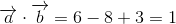The two vectors are not orthogonal.

### Example Question #17 : Normal Vectors

Determine whether the two vectors,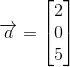and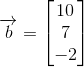, are orthogonal or not.

The two vectors are not orthogonal.

The two vectors are orthogonal.

The two vectors are not orthogonal.

Explanation:

Vectors can be said to be orthogonal, that is to say perpendicular or normal, if their dot product amounts to zero:To find the dot product of two vectors given the notationSimply multiply terms across rows:For our vectors,and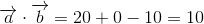The two vectors are not orthogonal.

### Example Question #18 : Normal Vectors

Determine whether the two vectors,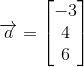and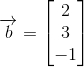, are orthogonal or not.

The two vectors are orthogonal.

The two vectors are not orthogonal.

The two vectors are orthogonal.

Explanation:

Vectors can be said to be orthogonal, that is to say perpendicular or normal, if their dot product amounts to zero:To find the dot product of two vectors given the notationSimply multiply terms across rows:For our vectors,and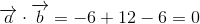The two vectors are orthogonal.

### Example Question #19 : Normal Vectors

Determine whether the two vectors,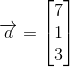and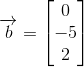, are orthogonal or not.

The two vectors are not orthogonal.

The two vectors are orthogonal.

The two vectors are not orthogonal.

Explanation:

Vectors can be said to be orthogonal, that is to say perpendicular or normal, if their dot product amounts to zero:To find the dot product of two vectors given the notationSimply multiply terms across rows:For our vectors,and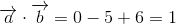The two vectors are not orthogonal.

### Example Question #20 : Normal Vectors

Determine whether the two vectors,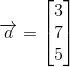and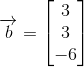, are orthogonal or not.

The two vectors are orthogonal.

The two vectors are not orthogonal.

The two vectors are orthogonal.

Explanation:

Vectors can be said to be orthogonal, that is to say perpendicular or normal, if their dot product amounts to zero:To find the dot product of two vectors given the notationSimply multiply terms across rows:For our vectors,and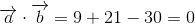The two vectors are orthogonal.